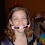## Thursday, March 22, 2007

### Five Balls Are Removed

A hat contains a number N of blue balls and red balls. If five balls are removed randomly from the hat, the probability is precisely 1/2 that all five balls are blue. What is the smallest value of N for which this is possible.

1.For the smallest value of N possible, the numbers of blue balls and total balls are going to have to be relatively close together. In fact, of all the combinations that would work, the smallest N would have to be the case where those two values are closest. We'll take the most extreme case to start and assume they are only one apart. Then, you get

P=(N-1)/N*(N-2)/(N-1)*(N-3)/(N-2)*(N-4)/(N-3)*(N-5)/(N-4)=1/2

for the probability that all five balls are blue (again we're assuming there are N total balls and N-1 blue balls, and each time we draw a blue ball, we subtract one from each). You can obviously see that everything cancels out except (N-5)/N=1/2, so N=10.

10 total balls, 9 blue balls.

2.I couldn't have said it better myself. So... I won't. ;-)

Nice work Abe!

Leave your answer or, if you want to post a question of your own, send me an e-mail. Look in the about section to find my e-mail address. If it's new, I'll post it soon.

Please don't leave spam or 'Awesome blog, come visit mine' messages. I'll delete them soon after.

Enter your Email and join hundreds of others who get their Question of the Day sent right to their mailbox

The Lamplight Manor Puzz 3-D